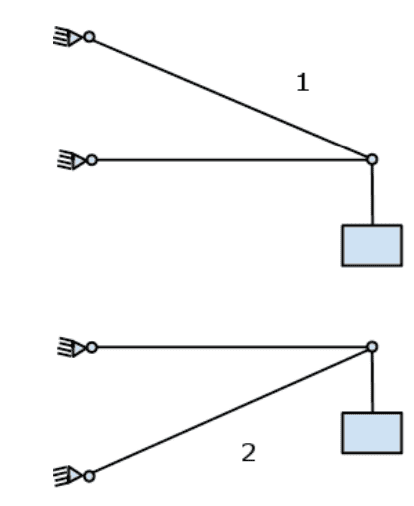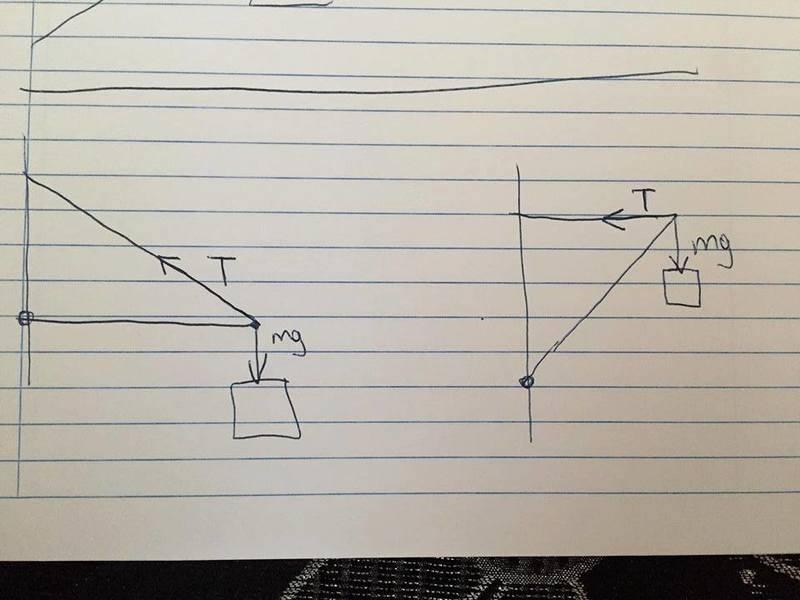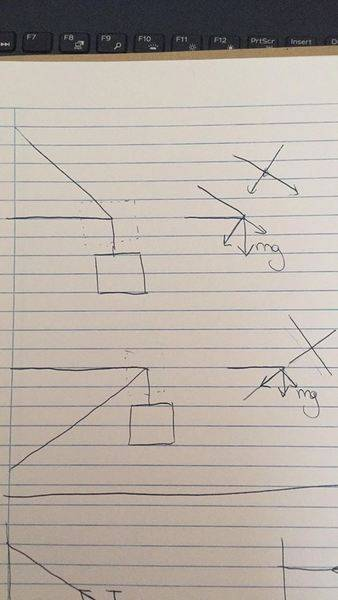# Mass suspended by bars in two configurations

• Pauleta
In summary, the mass in the first case will travel the most distance in the vertical direction assuming we are in the elastic region.f

#### Pauleta

New poster has been reminded to use the Homework Help Template in the schoolwork forumsI have these two cases of a mass hanging from beams. The question is in which of the two cases will the mass travel the most distance in the vertical direction assuming we are in the elastic region.

All i can think of is to calculate the tension for each of the two cases, Whichever case has the biggest tension will be the one that will travel the most in the vertical direction. But i am not sure

#### Attachments

Last edited by a moderator:
Welcome to the PF.

So each of the lines is a solid beam that has a finite modulus of elasticity? Can you show FBDs for all elements in each of the two diagrams? Thanks.

•Bystander
Whichever case has the biggest tension
You have a tension and a compression. It may be that one arrangement has the greater tension while the other has the greater compression.
Create unknowns for the elasticity etc. and figure out what the changes in length would be. You will need to assume small changes and see what they imply for the drop of the mass.

•berkeman
This is how i draw the FBD for the two cases (or what i believe it is) and based on those diagrams if i calculate the torques at the black dot my results show me that the Tension produced in case 1 is smaller than the Tension produced in case 2 and based this result i thought that since the tension is smaller the distance will be smaller#### Attachments

This is how i draw the FBD for the two cases (or what i believe it is) and based on those diagrams if i calculate the torques at the black dot my results show me that the Tension produced in case 1 is smaller than the Tension produced in case 2 and based this result i thought that since the tension is smaller the distance will be smaller

View attachment 227838
There are two things wrong with that reasoning.
As I mentioned, you are overlooking that the lower member will be in compression, so will shrink. This will also affect the height of the mass.
Also, a change in length of a member does not lead to the same change in the height of the mass. Indeed, in your right-hand diagram, stretching the upper member a little will make no difference to the height of the mass.

•berkeman
There are two things wrong with that reasoning.
As I mentioned, you are overlooking that the lower member will be in compression, so will shrink. This will also affect the height of the mass.
Also, a change in length of a member does not lead to the same change in the height of the mass. Indeed, in your right-hand diagram, stretching the upper member a little will make no difference to the height of the mass.

I think i am missing something fundamental here. Are you saying there is no straight-forward solution to that problem or is it that i am oversimplifying it?

What if i draw the FBD in a different coordinate system without taking into account the tension. Just analysing the mass in the 2 cases. If i do it like that i can see that in the first case the mass creates tension whereas in the other case the mass creates compression to the longer beam.#### Attachments

i am oversimplifying it?
Yes.
in the first case the mass creates tension whereas in the other case the mass creates compression to the longer beam.
If you draw the full FBDs you will see that in both cases the upper is under tension and the lower is under compression.